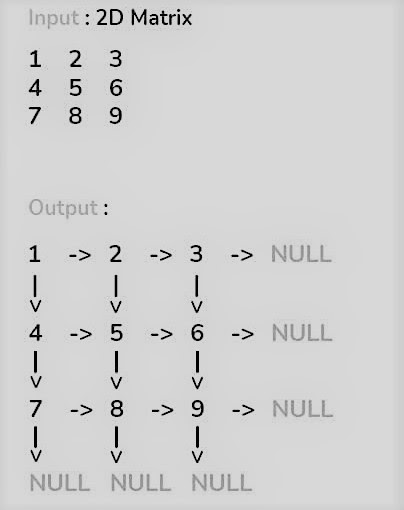# Construct a Linked List from a 2D Matrix

## Introduction

The linked list is one of the most important concepts and data structures to learn.

This problem of constructing a linked list from a 2D matrix will cover a basic understanding of the linked list.

## Problem Statement

Given a 2D matrix, construct a linked list from the matrix. So, we need to represent the 2D matrix as a linked list.## Method

We need to create a node for each cell of the matrix and then attach it to its right and down elements using the right and down pointers of the node, respectively.

So, we need to construct a linked list in which each node will have two pointers named right and down.

When we reach the boundary of the matrix, point to a NULL pointer.

The idea is to create m linked lists ( where m is the number of rows in the matrix) whose each node stores its right node. The head pointers of each m linked list are stored in an array of nodes.

Then, traverse m lists, for every ith and (i+1)th list, set the down pointers of each node of ith list to its corresponding node of (i+1)th list.

Algorithm

• Create an array of node pointers (say head) of size equal to the number of rows(m) in the 2D matrix.
• At first, traverse through all the rows of the matrix, and inside this for loop initialize the head[i] to NULL.
• Create a nested for loop to traverse the column elements of the matrix and, while traversing, create new nodes having a value equal to the value at the corresponding position in the given matrix and then check if head[i] is NULL or not.
• If head[i] is NULL, then we are at the first column, so we need to store the address of the newly created node in the head[i].

• If head[i] is not NULL, we will connect the current node in the ith linked list using the right pointer.

• Then store the address of this node in the righttemp node pointer so that we can connect the new node after this node further.

• After the above nested for loops, we need to loop through the head pointers' array again and connect down pointers of the corresponding ith linked list to (i+1)th linked list.
•  Return head, as it would contain the address of the first node of the newly created linked list.

Output

## Complexity analysis

The time complexity of the above algorithm is O(m*n) in the worst case where m is no. of rows in the matrix and n is the number of columns.

Space complexity is also O(m*n) as we are creating m*n number of nodes.

## FAQs

A linked list is a dynamic data structure where each element (called a node) is made up of two items: the data and a reference (or pointer), which points to the next node. A linked list is a collection of nodes where each node is connected to the next node through its pointer.

2). How many types of linked lists are there?

There are mainly four types of linked lists.

## Key Takeaways

This article covered how to construct a linked list from a 2D matrix. Having a good grasp of Linked Lists can be a huge plus point in a coding interview.

Check out the CodeStudio library for getting a better hold of the data structures and algorithms.

Side by side, you can also practice a wide variety of coding questions commonly asked in interviews in CodeStudio. Along with coding questions, you can also find the interview experience of scholars working in renowned product-based companies here.

Happy learning!